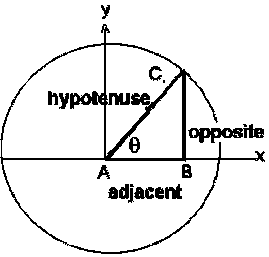# Trigonometry Functions Calculator

Calculate the values for six possible trigonometric functions or ratios as sine, cosine, tangent, cotangent, secant and cosecant against selection.

Trigonometry Value:

 Sin(x): Cos(x): Tan(x): cosec(x): Sec(x): Cot(x):

## The formulas used:

Sinθ = 1 / Cosecθ

Cosθ = 1 / Secθ

Tanθ = Sinθ / Cosθ

Cosecθ = 1 / Sinθ

Secθ = 1 / Cosθ

Cotθ = 1 / TanθThe trigonometric functions are functions of an angle and side measurement. It is relate the angles of a triangle to the lengths of its sides.
The summarized table for trigonometric functions and important Formula as follows: